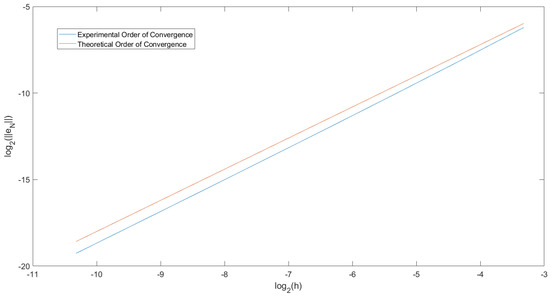Previous Article in Journal
Semi-Local Convergence of a Seventh Order Method with One Parameter for Solving Non-Linear Equations
Previous Article in Special Issue
On Λ-Fractional Differential Equations

Article

# Detailed Error Analysis for a Fractional Adams Method on Caputo–Hadamard Fractional Differential Equations

Department of Physical, Mathematical and Engineering, University of Chester, Chester CH1 4BJ, UK
*
Author to whom correspondence should be addressed.
These authors contributed equally to this work.
Academic Editor: Sotiris K. Ntouyas
Foundations 2022, 2(4), 839-861; https://doi.org/10.3390/foundations2040057
Received: 28 August 2022 / Revised: 12 September 2022 / Accepted: 13 September 2022 / Published: 22 September 2022
(This article belongs to the Special Issue Recent Advances in Fractional Differential Equations and Inclusions)
We consider a predictor–corrector numerical method for solving Caputo–Hadamard fractional differential equation over the uniform mesh $\mathrm{log}{t}_{j}=\mathrm{log}a+\left(\mathrm{log}\frac{{t}_{N}}{a}\right)\left(\frac{j}{N}\right),\phantom{\rule{0.166667em}{0ex}}j=0,1,2,\dots ,N$ with $a\ge 1$, where $\mathrm{log}a=\mathrm{log}{t}_{0}<\mathrm{log}{t}_{1}<\dots <\mathrm{log}{t}_{N}=\mathrm{log}T$ is a partition of $\left[\mathrm{log}a,\mathrm{log}T\right]$. The error estimates under the different smoothness properties of the solution y and the nonlinear function f are studied. Numerical examples are given to verify that the numerical results are consistent with the theoretical results. View Full-Text
Show FiguresFigure 1

MDPI and ACS Style

Green, C.W.H.; Yan, Y. Detailed Error Analysis for a Fractional Adams Method on Caputo–Hadamard Fractional Differential Equations. Foundations 2022, 2, 839-861. https://doi.org/10.3390/foundations2040057

AMA Style

Green CWH, Yan Y. Detailed Error Analysis for a Fractional Adams Method on Caputo–Hadamard Fractional Differential Equations. Foundations. 2022; 2(4):839-861. https://doi.org/10.3390/foundations2040057

Chicago/Turabian Style

Green, Charles Wing Ho, and Yubin Yan. 2022. "Detailed Error Analysis for a Fractional Adams Method on Caputo–Hadamard Fractional Differential Equations" Foundations 2, no. 4: 839-861. https://doi.org/10.3390/foundations2040057

Find Other Styles

1